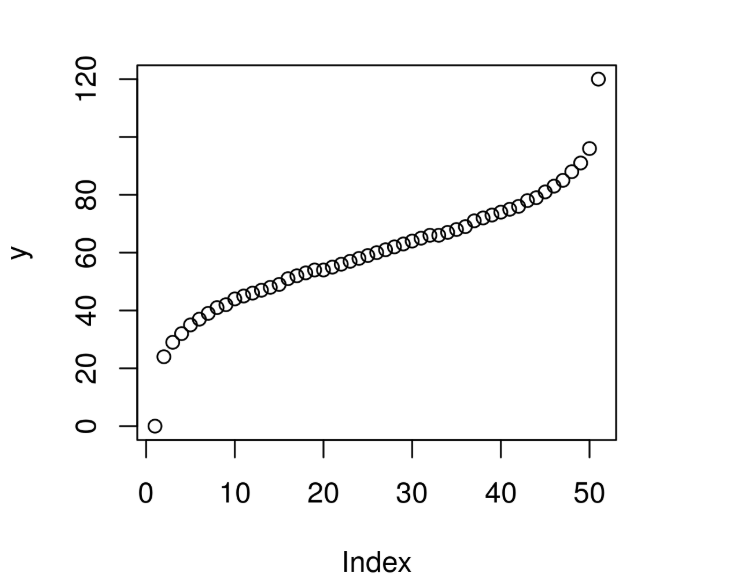Related Articles
Compute the value of Quantile Function over Wilcoxon Signedrank Distribution in R Programming – qsignrank() Function
• Last Updated : 25 Jun, 2020

`qsignrank()` function in R Language is used to compute the value of Quantile Function(QF) over Wilcoxon Signedrank Statistic Distribution for a sequence of Numeric values.

Syntax: qsignrank(x, n)

Parameters:
x: Numeric Vector
n: Sample Size

Example 1:

 `# R Program to compute the value of``# QF over Wilcoxon Signedrank Distribution`` ` `# Creating a sequence of x-values``x <``-` `seq(``0``, ``1``, by ``=` `0.1``)`` ` `# Calling qsignrank() Function``y <``-` `qsignrank(x, n ``=` `10``)``y`

Output:

```   0 15 19 22 25 27 30 33 36 40 55
```

Example 2:

 `# R Program to compute the value of``# QF over Wilcoxon Signedrank Distribution`` ` `# Creating a sequence of x-values``x <``-` `seq(``0``, ``1``, by ``=` `0.02``)`` ` `# Calling qsignrank() Function``y <``-` `qsignrank(x, n ``=` `15``)`` ` `# Plot a graph``plot(y)`

Output:Attention reader! Don’t stop learning now. Get hold of all the important DSA concepts with the DSA Self Paced Course at a student-friendly price and become industry ready.

My Personal Notes arrow_drop_up